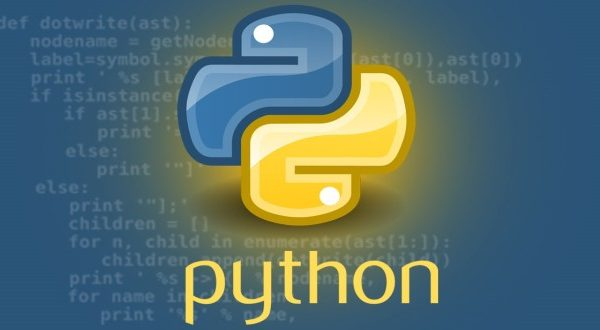# Matplotlib Box Plot – Tutorial and Examples### Introduction

There are many data visualization libraries in Python, yet Matplotlib is the most popular library out of all of them. Matplotlib’s popularity is due to its reliability and utility – it’s able to create both simple and complex plots with little code. You can also customize the plots in a variety of ways.

In this tutorial, we’ll cover how to plot Box Plots in Matplotlib.

Box plots are used to visualize summary statistics of a dataset, displaying attributes of the distribution like the data’s range and distribution.

### Importing Data

To create a Box Plot, we’ll need some data to plot. We’ll need to choose a dataset that contains continuous variables as features, since Box Plots visualize continuous variable distribution. We’ll be working with the Wine Quality dataset.

We’ll begin by importing all the libraries that we need. We’ll import